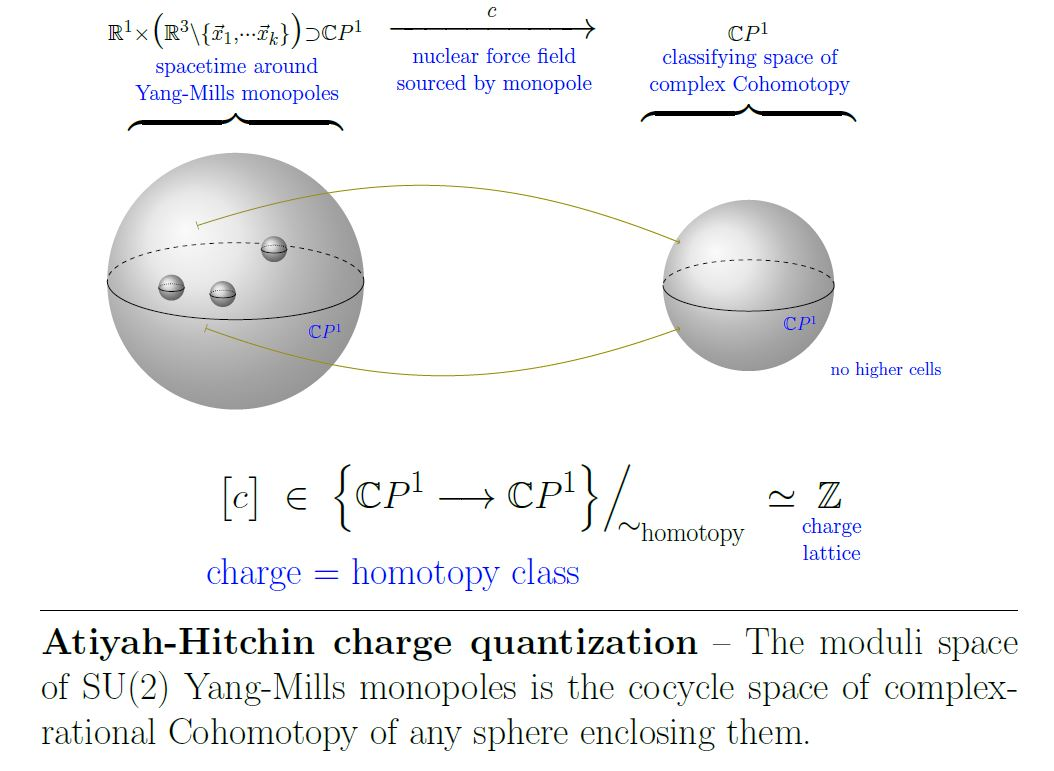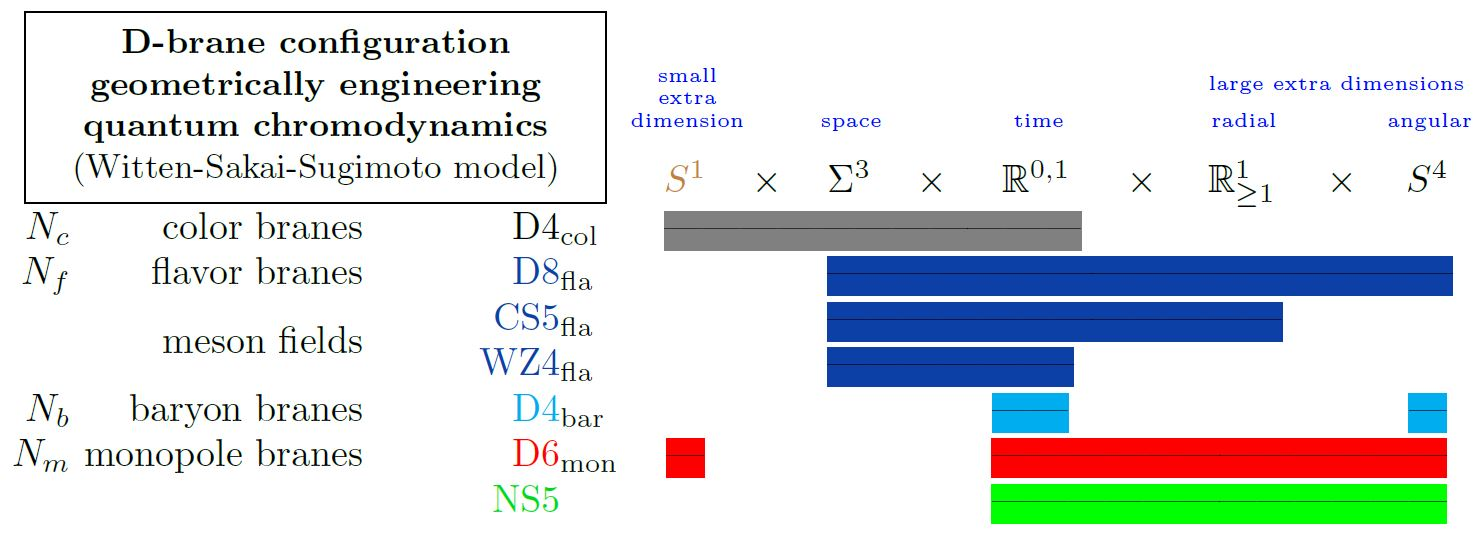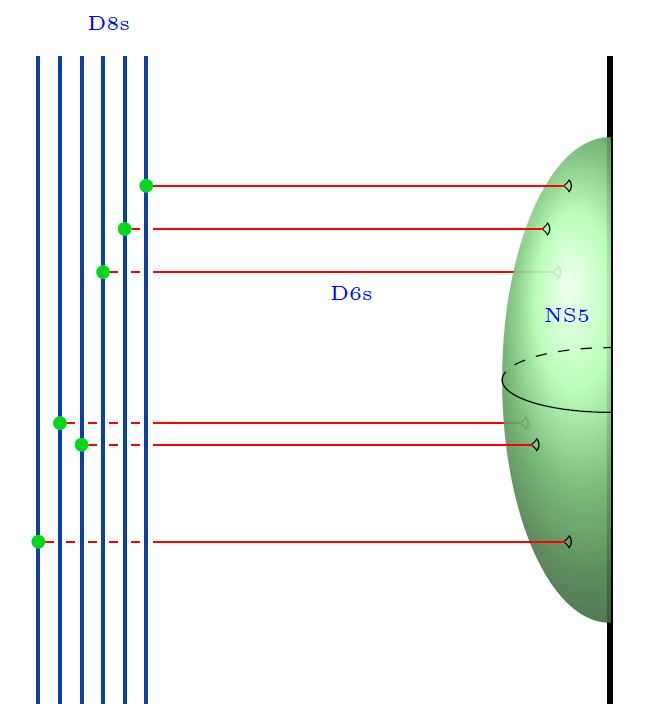# nLab moduli space of monopoles

Contents

### Context

#### Chern-Weil theory

∞-Chern-Weil theory

∞-Chern-Simons theory

∞-Wess-Zumino-Witten theory

# Contents

## Idea

By the Nahm transform, the moduli space of $x^4$-translation invariant self-dual Yang-Mills theory solitons on 4d Euclidean space $\mathbb{R}^{4}$ is equivalently the space of solutions to the Bogomolny equations on 3d Euclidean space, which in turn may be thought of as magnetic monopoles in 3d Euclidean Yang-Mills theory coupled to a charged scalar field (a “Higgs field”). Therefore this moduli space is traditionally referred to simply as the moduli space of magnetic monopoles (e.g. Atiyah-Hitchin 88) or just the moduli space of monopoles.

## Definition

The moduli space

(1)$\mathcal{M}_k \;\coloneqq\; \cdots$

of $k$ monopoles is … (Atiyah-Hitchin 88, p. 15-16).

## Properties

### Scattering amplitudes of monopoles

Write

(2)$Maps_{cplx\,rtnl}^{\ast/}\big( \mathbb{C}P^1, \mathbb{C}P^1 \big)_k \;\subset\; Maps_{cplx\,rtnl}^{\ast/}\big( \mathbb{C}P^1, \mathbb{C}P^1 \big) \;\subset\; Maps^{\ast/}\big( S^2, S^2 \big)$

for the space of pointed rational functions from the Riemann sphere to itself, of degree $k \in \mathbb{N}$, inside the full Cohomotopy cocycle space. The homotopy type of this mapping space is discussed in Segal 79, see homotopy of rational maps.

To each configuration $c \in \mathcal{M}_k$ of $k \in \mathbb{N}$ magnetic monopoles is associated a scattering amplitude

(3)$S(c) \in Maps_{cplx\,rtnl}^{\ast/}\big( \mathbb{C}P^1, \mathbb{C}P^1 \big)_k$

### Charge quantization in Cohomotopy

###### Proposition

(moduli space of k monopoles is space of degree $k$ complex-rational functions from Riemann sphere to itself)

The assignment (3) is a diffeomorphism identifying the moduli space (1) of $k$ magnetic monopoles with the space (2) of complex-rational functions from the Riemann sphere to itself, of degree $k$ (hence the cocycle space of complex-rational 2-Cohomotopy)

$\mathcal{M}_k \; \underoverset{ \simeq_{diff} }{ S }{ \;\;\longrightarrow\;\; } \; Maps_{cplx\,rtnl}^{\ast/} \big( \mathbb{C}P^1, \mathbb{C}P^1 \big)_k$

###### Proposition

(space of degree $k$ complex-rational functions from Riemann sphere to itself is k-equivalent to Cohomotopy cocycle space in degree $k$)

The inclusion of the complex rational self-maps maps of degree $k$ into the full based space of maps of degree $k$ (hence the $k$-component of the second iterated loop space of the 2-sphere, and hence the plain Cohomotopy cocycle space) induces an isomorphism of homotopy groups in degrees $\leq k$ (in particular a k-equivalence):

$Maps_{cplx\,rtnl}^{\ast/} \big( \mathbb{C}P^1, \mathbb{C}P^1 \big)_k \; \underset{ \simeq_{\leq k} }{ \;\;\hookrightarrow\;\; } \; Maps^{\ast/}\big( S^2, S^2 \big)_k$

Hence, Prop. and Prop. together say that the moduli space of $k$-monopoles is $k$-equivalent to the Cohomotopy cocycle space $\mathbf{\pi}^2\big( S^2 \big)_k$.

$\mathcal{M}_k \; \underoverset{ \simeq_{diff} }{ S }{ \;\;\longrightarrow\;\; } \; Maps_{cplx\,rtnl}^{\ast/} \big( \mathbb{C}P^1, \mathbb{C}P^1 \big)_k \; \underset{ \simeq_{\leq k} }{ \;\;\hookrightarrow\;\; } \; Maps^{\ast/}\big( S^2, S^2 \big)_k$

This is a non-abelian analog of the Dirac charge quantization of the electromagnetic field, with ordinary cohomology replaced by Cohomotopy cohomology theory:

$\,$### Relation to braid groups

###### Proposition

(moduli space of monopoles is stably weak homotopy equivalent to classifying space of braid group)

For $k \in \mathbb{N}$ there is a stable weak homotopy equivalence between the moduli space of k monopoles (?) and the classifying space of the braid group $Braids_{2k}$ on $2 k$ strands:

$\Sigma^\infty \mathcal{M}_k \;\simeq\; \Sigma^\infty Braids_{2k}$

### Geometric engineering by D$p$-D$(p+2)$-brane intersections

Generally Dp-D(p+2)-brane intersections geometrically engineer Yang-Mills monopoles in the worldvolume of the higher dimensional D$(p+2)$-branes.

Specifically for $p = 6$, i.e. for D6-D8-brane intersections, this fits with the Witten-Sakai-Sugimoto model geometrically engineering quantum chromodynamics, and then gives a geometric engineering of the Yang-Mills monopoles in actual QCD (HLPY 08, p. 16).graphics from Sati-Schreiber 19c

Here we are showing

1. the color D4-branes;

2. the flavor D8-branes;

with

1. the 5d Chern-Simons theory on their worldvolume

2. the corresponding 4d WZW model on the boundary

both exhibiting the meson fields

3. (see below at WSS – Baryons)

4. (see at D6-D8-brane bound state)

5. the NS5-branes.

### General

On the ordinary cohomology of the moduli space of YM-monopoles:

The special case of gauge group SU(3):

### Identification of Yang-Mills monopoles with rational maps

The following lists references concerned with the identification of the (extended) moduli space of Yang-Mills monopoles (in the BPS limit, i.e. for vanishing Higgs potential) with a mapping space of complex rational maps from the complex plane, equivalently holomorphic maps from the Riemann sphere $\mathbb{C}P^1$ (at infinity in $\mathbb{R}^3$) to itself (for gauge group SU(2)) or generally to a complex flag variety such as (see Ionnadou & Sutcliffe 1999a for review) to a coset space by the maximal torus (for maximal symmetry breaking) or to complex projective space $\mathbb{C}P^{n-1}$ (for gauge group SU(n) and minimal symmetry breaking).

The identification was conjectured (following an analogous result for Yang-Mills instantons) in:

Full understanding of the rational map involved as “scattering data” of the monopole is due to:

The identification with (pointed) holomorphic functions out of $\mathbb{C}P^1$ was proven…

…for the case of gauge group $SU(2)$ (maps to $\mathbb{C}P^1$ itself) in

…for the more general case of classical gauge group with maximal symmetry breaking (maps to the coset space by the maximal torus) in:

… for the fully general case of semisimple gauge groups with any symmetry breaking (maps to any flag varieties) in

and for un-pointed maps in

Further discussion:

Review:

• Alexander B. Atanasov, Magnetic monopoles and the equations of Bogomolny and Nahm (pdf), chapter 5 in: Magnetic Monopoles, ‘t Hooft Lines, and the Geometric Langlands Correspondence, 2018 (pdf, slides)

On the relevant homotopy of rational maps (see there for more references):

### As transversal D$p$/D$(p+2)$-brane intersections

For transversal D1-D3-brane bound states:

For transversal D2-D4 brane intersections (with an eye towards AdS/QCD):

• Alexander Gorsky, Valentin Zakharov, Ariel Zhitnitsky, On Classification of QCD defects via holography, Phys. Rev. D79:106003, 2009 (arxiv:0902.1842)

For transversal D3-D5 brane intersections:

For transversal D6-D8-brane intersections (with an eye towards AdS/QCD):

• Deog Ki Hong, Ki-Myeong Lee, Cheonsoo Park, Ho-Ung Yee, Section V of: Holographic Monopole Catalysis of Baryon Decay, JHEP 0808:018, 2008 (https:arXiv:0804.1326)

With emphasis on half NS5-branes in type I' string theory:

The moduli space of monopoles appears also in the KK-compactification of the M5-brane on a complex surface (AGT-correspondence):

• Benjamin Assel, Sakura Schafer-Nameki, Jin-Mann Wong, M5-branes on $S^2 \times M_4$: Nahm’s Equations and 4d Topological Sigma-models, J. High Energ. Phys. (2016) 2016: 120 (arxiv:1604.03606)

### As Coulomb branches of $D=3$$\mathcal{N}=4$ SYM

Identification of the Coulomb branch of D=3 N=4 super Yang-Mills theory with the moduli space of monopoles in Yang-Mills theory:

### Rozansky-Witten invariants

Discussion of Rozansky-Witten invariants of moduli spaces of monopoles:

### Relation to braids

Relation to braid groups:

Relation of Dp-D(p+2)-brane bound states (hence Yang-Mills monopoles) to Vassiliev braid invariants via chord diagrams computing radii of fuzzy spheres: